시간 제한메모리 제한제출정답맞힌 사람정답 비율
1 초 1024 MB6511100.000%

## 문제

A convex-hull of a set of 2-dimensional points P is defined as the polygon with the smallest perimeter that encloses all points in P. It is “convex” because such a polygon will always have a convex shape.

You are given a set P that contains N points. For each point (xi, yi) ∈ P, you should output the number of points in P \ {(xi , yi)} that lies exactly at the convex-hull enclosing all points in P \ {(xi, yi)}. Note that the notation P \ {(xi, yi)} means that the point (xi, yi) is taken out from set P. In other words, the ith point is taken out from P when the convex-hull in consideration is built. Also note that the constructed convex-hull can also degenerate into a line or a point depends on the set of points in consideration.

Consider the following example. Let there be N = 5 points: (1, 0), (5, 0), (7, 0), (3, 4), and (3, 2).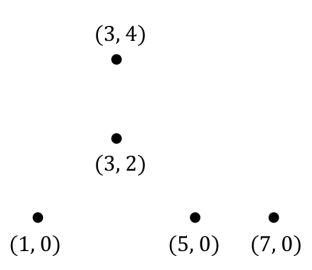The set of all points P.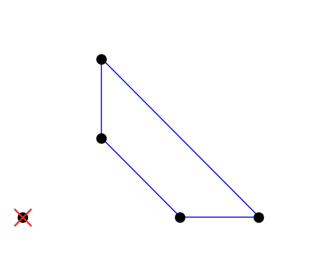The convex-hull when P1 = (1, 0) is removed. There are 4 points that lies at the convex-hull.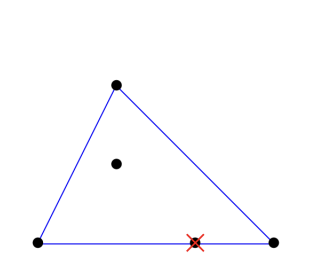The convex-hull when P2 = (5, 0) is removed. There are 3 points that lies at the convex-hull.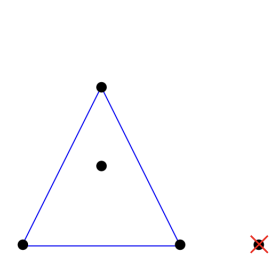The convex-hull when P3 = (7, 0) is removed. There are 3 points that lies at the convex-hull.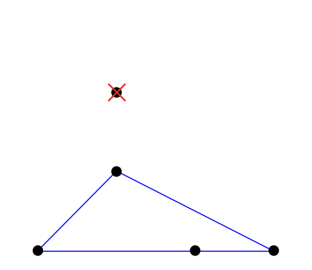The convex-hull when P4 = (3, 4) is removed. There are 4 points that lies at the convex-hull.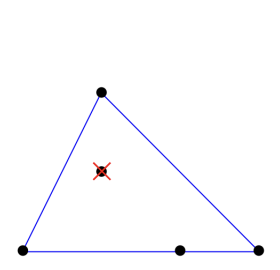The convex-hull when P5 = (3, 2) is removed. There are 4 points that lies at the convex-hull.

## 입력

Input begins with a line containing an integer N (2 ≤ N ≤ 100 000) representing the number of points in the set P. The next N lines each contains two integers xi yi (−109 ≤ xi, yi ≤ 109) representing the (x, y) coordinate of the ith point. You are guaranteed that there are no two points located at the same coordinate.

## 출력

Output contains N lines. The ith line contains an integer representing the number of points in P \ {(xi, yi)} that lies exactly at the convex-hull of P \ {(xi, yi)}.

## 예제 입력 1

5
1 0
5 0
7 0
3 4
3 2


## 예제 출력 1

4
3
3
4
4


## 예제 입력 2

3
0 0
0 100
100 0


## 예제 출력 2

2
2
2